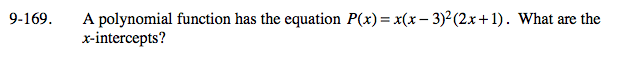### Home > A2C > Chapter 9 > Lesson 9.3.3 > Problem9-169

9-169.

A polynomial function has the equation P(x) = x(x − 3)2(2x + 1). What are the x-intercepts? Homework Help ✎(0, 0), (3, 0) and (−0.5, 0)